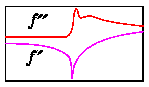# Choosing wavelengths for MAD data collection

Once you have determined the actual scattering factors f' and f'' in the energy range of interest for your sample crystal (see theory vs experiment), you need to choose exactly which x-ray wavelengths to use for MAD data collection.Schematic of experimental values for f' and f'' as a function of x-ray energy

## How many wavelengths to use?

For a single type of anomalous scattering atom, i.e. a MAD experiment at a single absorption edge, you need a minimum of 2 wavelengths (see MAD phasing tutorial). It is better to be have more data points so that the set of simultaneous equations for MAD phasing is over-determined, so 3 wavelengths are better than 2. Beyond that it is a question of diminishing returns; 4 is better than 3, but may not be worth the extra data collection time for the slight improvement.

## What should they be?

• The largest signal will come from choosing the wavelength with maximal f'' (1 in the figure above).
• The second wavelength is usually chosen to have maximal |f'| (2 in the figure above). Note that (1 and2) are very close together, requiring great precision in setting up the apparatus which controls wavelength during data collection.
• Additional wavelengths (3 and4) are chosen at points remote from the absorption edge. The available signal increasing slowly as the distance from the first two wavelengths increases. However the diffraction conditions (crystal absorption and diffracting power, diffraction geometry, etc) become more disparate as the distance increases. The choice usually comes down to the practical limitations imposed by the particular beamline apparatus being used. Typically3 and4 are between 100eV and 1000eV from the absorption edge.X-ray Anomalous ScatteringMAD phasing tutorial
Ethan A Merritt ©1996-2001/ merritt@u.washington.edu / Biomolecular Structure Center at UW